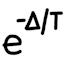#Simulated annealing demo

## Scenario

Simulated annealing is a powerful technique to optimize variables, especially in high dimensional spaces with thousands of variables. Just for fun, I wrote a program to experiment with annealing the pixels in a random image.

My program begins by generating a 256×256 image with uniformly random pixel values in RGB24 (i.e. rainbow noise). The simulated annealing process seeks to reduce the total “energy” in the entire image by swapping random adjacent pixels.

The energy in the image is defined as the sum of absolute differences between all horizontally and vertically adjacent pixels in all 3 color channels. For example, between two adjacent pixels with the colors (255,128,0) and (31,178,99), the energy is 373.

At each iteration, a random pixel in the image is picked, and it is swapped with either its right neighbor or bottom neighbor. The energy of the new image is calculated. The difference in energy, along with the current temperature, determines the probability that the swap is accepted. If rejected, the pixels are swapped back and the energy remains unchanged.

The current implementation has a simple cooling schedule where the temperature goes from startTemperature down to zero linearly. The probability of accepting a swap is the standard formula 2energyDiff / currentTemperature; this implies that reducing energy is always accepted.

(Note that because `Math.exp()` is rather slow (about 5 million calls per second on my desktop computer), I implemented a custom 2-to-the-power-of function which is reasonably accurate and much faster (~300 million per second). This does not affect the overall behavior of simulated annealing, because exp and 2-pow can be made equivalent by scaling the temperature by a constant factor.)

## Resulting images

Starting temperature
20 40 70 100 200 600 2000
Num iters Initial (zero) Original image
10 million Image Image Image Image Image Image Image
100 million Image Image Image Image Image Image Image
1 billion Image Image Image Image Image Image Image
10 billion Image Image Image Image Image Image Image
100 billion Image Image Image Image Image Image Image
1 trillion Image Image Image Image Image Image Image
10 trillion Image Image Image Image Image Image ImageDiscussion of results: We can see that as the number of iterations is increased, the annealed image becomes smoother and smoother. The starting temperature makes a big difference on the effectiveness of simulated annealing, however – too low and the process gets trapped in a local minimum early on, too high and the process doesn’t pursue the energy reduction aggressively enough. I empirically determined that a starting temperature of 100 seems to yield the best results.

## Live demo (JavaScript)

 Image size: squared Number of iterations: million Starting temperature: Current iterations: Current temperature: Current energy:

## Source code

Download:Notes:

• The Java version is recommended, because it’s easier and safer to work with. The C version is available for raw speed (about 2× faster), but it only runs on x86-64 due to the hand-written assembly code.

• To use the program, compile the source code, and run the executable with no arguments. It will print status messages to the standard error stream, and will write a BMP file to the current working directory upon completion of simulated annealing.

• Note that in the output messages, the “SwapDiff” and “AcceptProb” columns are just a sample of how much energy difference the current iteration is facing and its acceptance probability. The data does not represent a complete log of all the energy changes that have occurred in the simulation run.

• The image dimensions and simulated annealing parameters are hard-coded into the program’s code. If you want to play with different settings, you will need to tweak the code and recompile.

• The Java version is considered the reference version. The C version can be tweaked to match the output of the Java version, as long as RNG re-seeding is disabled and `MtRandom_next_int_bounded()` is tweaked.

• A straight port of the Java code to C seems to run at about the same speed; on my machine they’re each about 10 million iterations per second. I thought the C code would have a significant speed-up, but it doesn’t seem to be the case. This is why I spent the effort to augment the C code with optimized functions in x86 assembly language using SIMD instructions.

• The current simulated annealing experiment swaps a random pixel with its horizontal or vertical neighbor. If instead we swap two random pixels anywhere in the image, the result converges much faster, but the images look somewhat different – we get uniform-looking fractal mountains instead of the current ridges and holes. The results of this alternate experiment are not published, however.